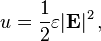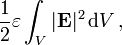# The EM energy of an electron

In another recent thread it was being discussed whether there is energy in the field of an electron according to $$U = \frac{1}{2}\epsilon_0 E^2.$$

I began by saying "yes", then changed to "no" because of the way the equation was derived. (It was derived by bringing together N=2 or more charges and ignoring the self-energy of each electron.)

I now wish to change my answer back to "yes", that the equation still works even for a single electron though the volume limits of integration (and therefore the exact value of the energy) is not known.

My argument is that it can be applied to the three quarks in the proton. From a great distance the proton an electron have the same charge and therefore you should be able to integrate the energy density of the electron according to the above equation at least up the exclusion of a sphere of protonic radius. The field energy of the electron should be at least this great and probably greater since it is smaller in size than the proton.

http://en.wikipedia.org/wiki/Electric_field#Energy_in_the_electric_field

The energy density of the electric field is given by:The total energy stored in the electric field in a given volume V is thereforeSee also teh section below that titled "Parallels between electrostatics and gravity"

this is for gravity but the equations are the same
http://en.wikipedia.org/wiki/Gravitational_binding_energy
http://scienceworld.wolfram.com/physics/SphereGravitationalPotentialEnergy.html